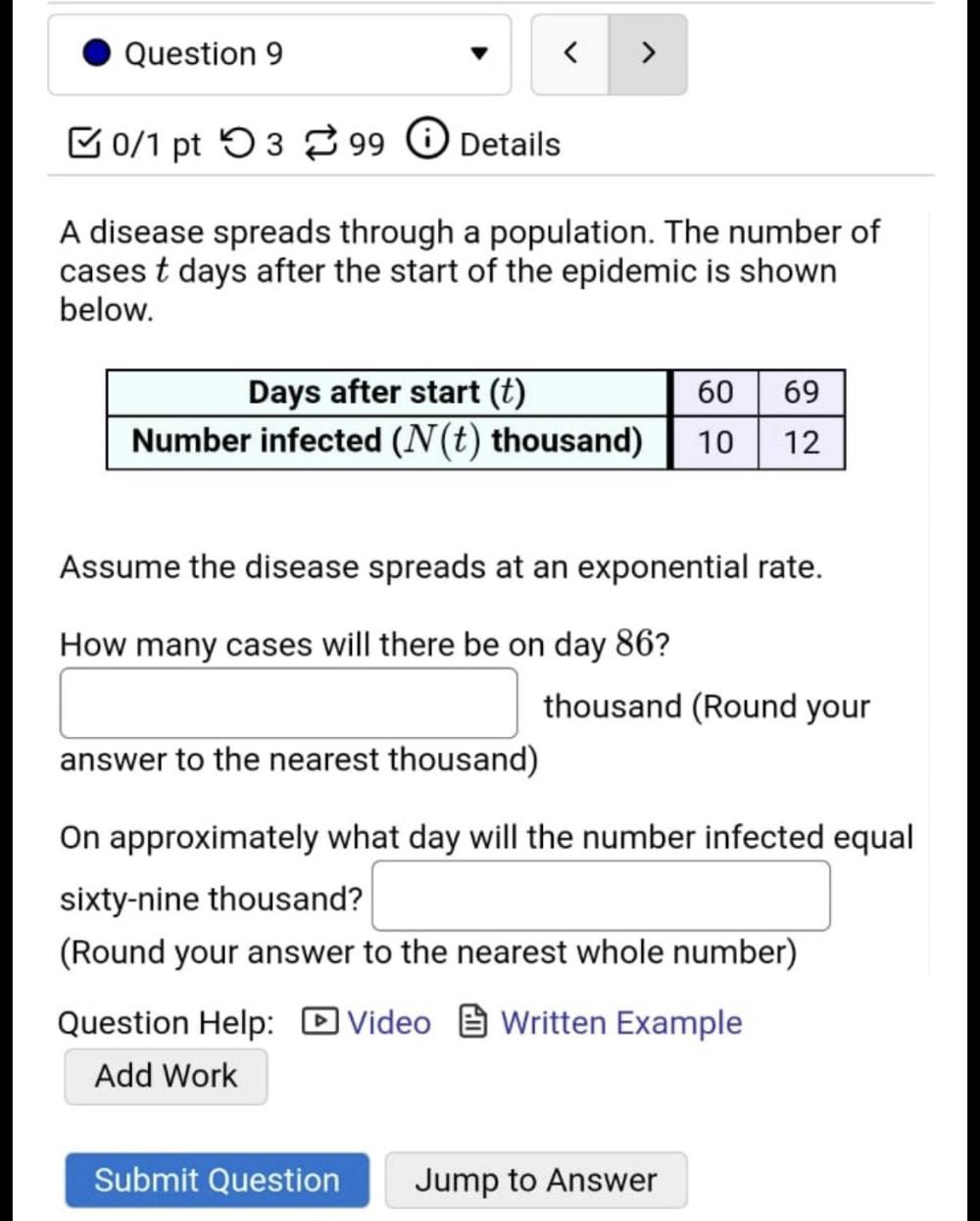Question:

# Question 9 < > B0/1 pt 53 99 0 Details A disease spreads through a population. The number of cases t days after the start of theQuestion 9 < > B0/1 pt 53 99 0 Details A disease spreads through a population. The number of cases t days after the start of the epidemic is shown below. Days after start (t) Number infected (N(t) thousand) 60 10 69 12 Assume the disease spreads at an exponential rate. How many cases will there be on day 86? thousand (Round your answer to the nearest thousand) On approximately what day will the number infected equal sixty-nine thousand? (Round your answer to the nearest whole number) Question Help: Video Written Example Add Work Submit Question Jump to Answer